# Derivatives, Part I: The Building Blocks

In the last post, we started with f(x) = x3 + 4x and by some mysterious process, we generated a new formula, f’(x) = 3x2 + 4. This “derivative” tells us the slope of the original function’s tangent lines.

As you enter AP Physics, I would like you to be able to find the derivatives of some basic functions.  I will let your math teachers explain where these formulas come from, but I want you to start getting familiar with these now.

Also, It is true that a TI-89 can find these derivative formulas for you.  But the ones I am asking you to learn are so frequently encountered that it would be a waste of time to have to reach for a calculator.  So let’s begin:

1. Constant Functions

Suppose f(x) = c, where c is a constant.  The graph of f will be a horizontal line.  The slope everywhere on that line is zero.  So our first rule is an easy one:

If f(x) = c then f'(x) = 0

2. Linear Functions

Suppose f(x) = kx, where k is a constant.  This graph will be a line passing through the origin with a slope, k.  So this rule is also straight-forward:

If f(x) = kx then f'(x) = k

3. The Power Rule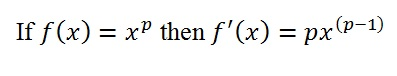Some examples:

f(x) = x2         →       f’(x) = 2x

f(x) = x3         →        f’(x) = 3x2

f(x) = x4         →        f’(x) = 4x3

4. Power Rule, Special Cases

The power rule works for negative powers and for fractional powers.  So you can use it to figure out the next two examples.  But I think these two are worth memorizing so that you don’t have to stop to re-derive them…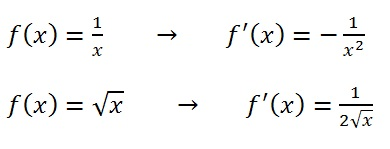5. Two Trig Functions

Your math teacher will teach you the derivatives of the functions for all six trigonometric ratios (and their inverse functions!) but for now, I’m just asking you to learn these two:

f(x) = sin(x)   →        f’(x) = cos(x)

f(x) = cos(x)   →        f’(x) = -sin(x)

If you would like to be convinced that the derivative of the sine function is the cosine function, play the video.

Watch the tangent line surf along the sine wave (blue).  Its slope changes in a pattern that matches the values of the cosine function (pink).

6. One more interesting function

There are many, many applications of exponential growth and decay in physics.  Exponential functions are in the form: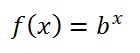where b is the base and x is the exponent.

Here is the graph for the case when b = 2:

As long as the base is greater than one, exponential functions will all have this same characteristic shape.

There is, however, one special value for the base: the Euler number, e = 2.7182…

I sometimes think of e as π’s neglected cousin.  They are both “transcendental” numbers, but I don’t know of any middle school that holds competitions for memorizing the digits of e. That may be because π is easier to explain:  it’s the ratio of a circle’s circumference to its diameter.

But what is e?  That’s a longer story (maybe for a future post — though there are entire books devoted to this subject).  For now, I will tell you an interesting and important fact about e.  The exponential function that has e as its base has a special property:

At every point along the graph of the function f(x) = ex, the slope of the tangent line at that point is equal to the value of the function at that same point.

Stop the movie at any point.  Compare the value of the function to the slope of the tangent line until you can say “Aha!”

This gives us one more derivative rule:

Enough to Build on…

The basic rules you have seen in this post will get us through most of AP Physics.  Now we have to learn how to apply these rules to combinations of functions.  That’s coming next.  For now, I’ll close by re-listing the rules.  Memorize them.  Print them out and put them in your notebook.  Know them like you know your times-tables.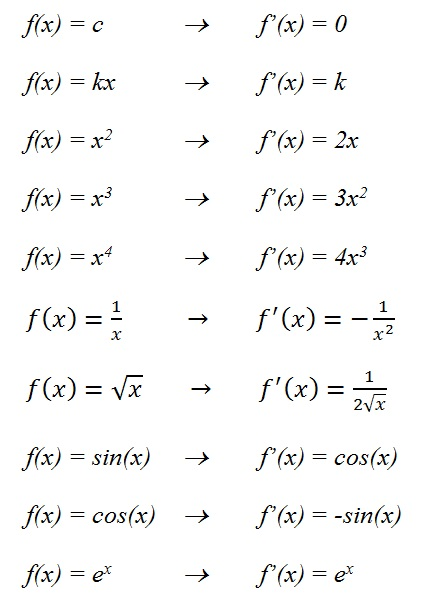# Slope-Finding Functions

By the end of this post, you will know what a derivative is.  You won’t know how to find one yet, but you will know what you have if you are given one!

Let’s say that we were given a function such as f(x) = x3 -4x.

We know it isn’t a linear function so when we graph it, we are not surprised to see the curves.  And if you can imagine a tangent line “surfing” along the curve, the slope of the tangent line would clearly be changing as you went along.  Here’s what I mean:

If you wanted to know the slope at a given point, you could estimate it by drawing the tangent line and then finding the slope of that tangent line (as we have seen in the last post).

But there is an alternative to that method that is useful and quick.  When you have the rule for a function, there is a way to derive a formula for a new function that will give you the slope of the original function’s tangent line at any x-value you choose.  Since it is derived from the original function, this new function is called “the derivative” of the original function.

For example, if the original function is:

f(x) = x3 – 4x

The derivative is written:

f’(x) = 3x2 – 4

The symbol f’(x) is pronounced “f-prime of x” and it is the most commonly used symbol to represent the derivative of the function f.

TWO THINGS YOU SHOULD NOT WORRY ABOUT (YET)

1. How did we get from the function f(x) = x3 – 4x to its derivative f’(x) = 3x2 – 4?

I will show you how to “take a derivative” in the next post.  Once you learn a handful of rules, the process is not difficult.  It’s easier than factoring.  It’s easier than long division.

2.  Why would anyone want a slope-finding formula ?

Once you know how to take derivatives, I can show you a variety of ways that they are useful.  In fact, you’ll even get to use them forward and backwards! (That was supposed to be some fore-shadowing…)

For now, my goal is simpler.  I just want to be sure that we understand what it is that the derivative formula tells us.  And once again…it’s better with diagrams.

I made the graphs and videos in this post using a web-based applet written by Paul Seeburger, a professor of mathematics at Monroe Community College in New York.  You can find the applet at:

http://web.monroecc.edu/manila/webfiles/pseeburger/JavaCode/DerivativeDemo2.htm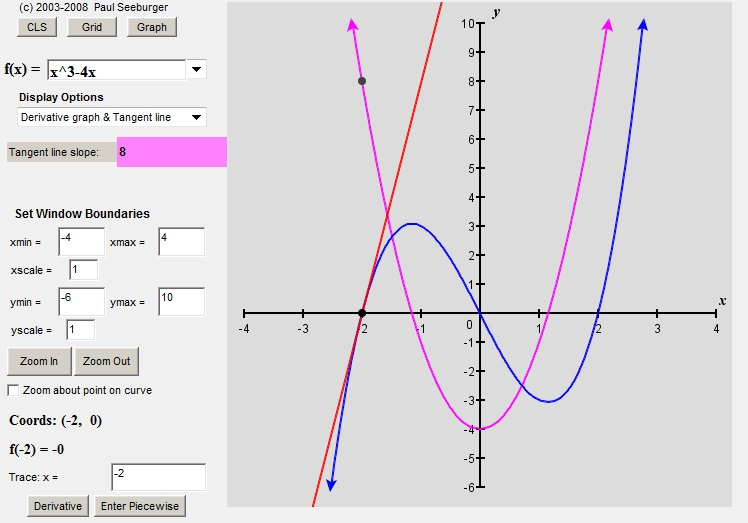The blue line is the original function, f(x) = x3 – 4x

The purple line is the derivative, f’(x) = 3x2 – 4.

The red line is the tangent line.  You can calculate the slope of that tangent using rise over run if you want to, but you don’t have to.  The applet does the calculation for you – look in the pink box.

Now let’s pick a point on the graph and examine all of the lines on this diagram.

At x = -2, f(-2) = (-2)3 – 4×(-2) = -8 + 8 = 0.  This means that (-2,0) is a point on the graph of f.

At that same point, the tangent line has a slope of 8 (as you can see in the pink box).

If we plug x = -2 into the derivative formula, we get f’(-2) = 3(-2)2 – 4 = 12 – 4 = 8.  And then, when you look at the graph of the derivative (in purple), you see that (2,8) is a point on that graph.  So the derivative formula tells us the slope – at least at that one point.  But does it always work?

Well, let’s look at another point:  x = -1.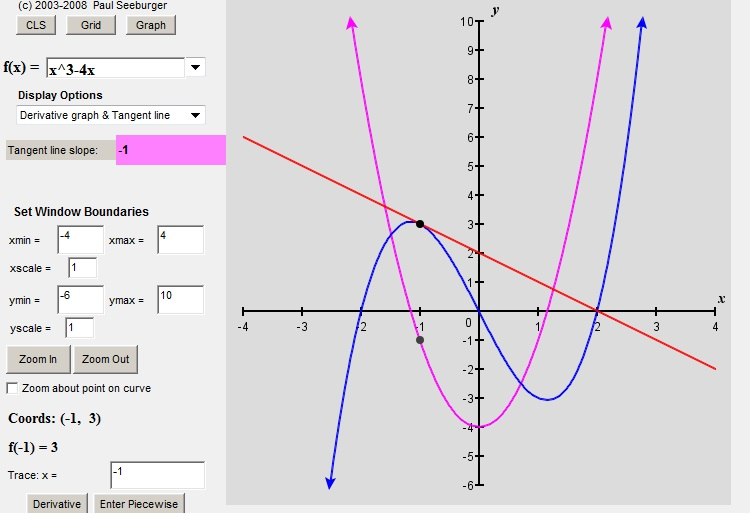(If you are playing along at home, you can enter any x-value you want into the box on the lower left hand side of the applet where it says “Trace x =”)

At x = -1, f(x) = (-1)3 – 4(-1) = 3.  So (-1, 3) is a point on the graph.

Then, f’(x) = 3(-1)2 – 4 = -1.  So we expect the slope of the tangent line to be -1, and it is. You can see that (-1,-1) is a point on the graph of the derivative (again, the purple one). So once again, the derivative formula has given the slope of the tangent line.

Here’s one more point that is worth noticing: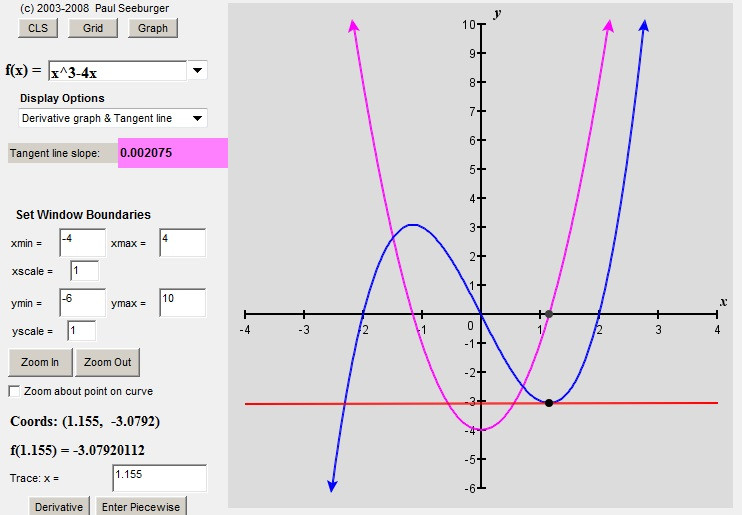Look at the value of the derivative.  And then look at the shape of the graph.  Where the derivative is zero, the graph has a horizontal tangent.  That little fact turns out to be a big part of one of the reasons why we care about derivatives. They help us to find places where the graph has a maximum or (as in this case) a minimum.  We’ll be hearing more about that later.

So we have confirmed, at least for the points we checked, that for this particular function, the derivative formula does in fact give values that match the slopes of the original function’s tangent lines.   If you want to, you can check many more points:

As you saw in the video above, Professor Seeburger’s applet lets you trace along the graph.  As you do, the tangent line surfs along the curve and the applet keeps track of all of the values.  Here is that video again, this time with the derivative function and the values all visible:

So if you are skeptical, run the video and then pause it wherever you want.  You’ll see that the slope of the tangent line always matches the value given by the derivative.  Derivatives really are slope-finding formulas.

# Velocity NOW!

When we examine the graph of your accelerating hair growth, it’s easy to show that the growth rate is increasing.  We can examine average growth rates for successive time intervals.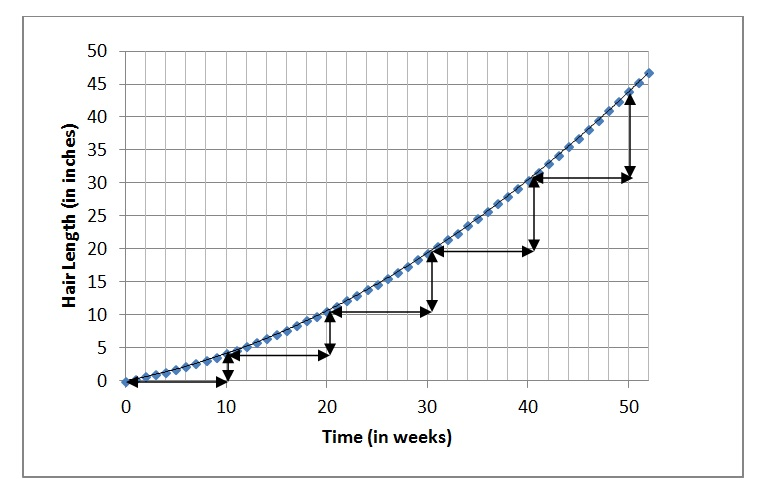For example, we could choose to look at 10-week intervals, calculating rise over run for each interval.  You should check for yourself to see that the average growth rate increased from just under 0.5 inches/week over the first 10-week interval to nearly triple that rate over the fifth 10-week interval.

Those are still just average rates.   But what if you wanted to know how fast your hair was growing right NOW!? Not over a finite interval but at a specific moment.   There is a way to answer that question too.  But you may have philosophical or mathematical objections.  “If no time passes, how can ANYTHING have a rate of change?”

The way we answer this kind of question is by making use of the idea of a limit.  Essentially, to find the slope at a given point, we start by finding the slope between two different points: the point we were asked about and another point a little later. But then we move that second point closer to the first one.  How close?  Reeeeeeeally close but not all the way there.  And as it gets closer, we look to see if there is a value that the slope approaches.  If so, we use that value as the instantaneous rate of change.

There are many websites that explore this concept in greater depth.  And they have some nice animations.  Here are a few:

http://clas.sa.ucsb.edu/staff/lee/Secant,%20Tangent,%20and%20Derivatives.htm

http://www.math.umn.edu/~garrett/qy/Secant.html

http://www.slu.edu/classes/maymk/Applets/SecantTangent.html

Here is an animation taken from the first website listed above: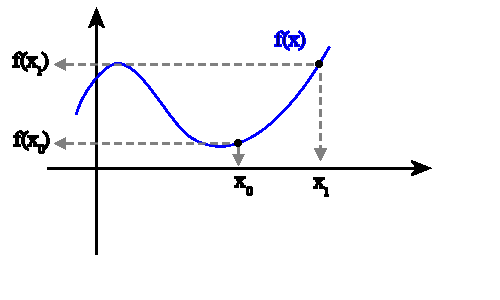UPDATE:  Now that I have discovered Paul Seeburger’s applet, here is a nicer look at that same idea:

I will leave most of the details for your math teachers to explain.  But I will point out that this is exactly why they want you to learn about limits.  Limits are the mathematical way to say “reeeeally close but not all the way there”.  It’s reasonable to say that limits were invented as a way of dealing with precisely this question: how do we find the slope of a line at a given point?

As your physics teacher, I want you to know two ways to deal with this question.

1. WITHOUT CALCULUS

Here is a graphical method that will give us an approximate answer:

(This is the method I use with my 11th graders.)

Use a ruler to draw a tangent line.  The slope of that tangent line is also the instantaneous rate of change.

So let’s say you wanted to how fast your hair was growing at the end of week #20.  (Note carefully: we are not looking for the average rate over the first 20 weeks.  We are looking for the instantaneous rate exactly at t = 20 weeks!    It’s a different question.)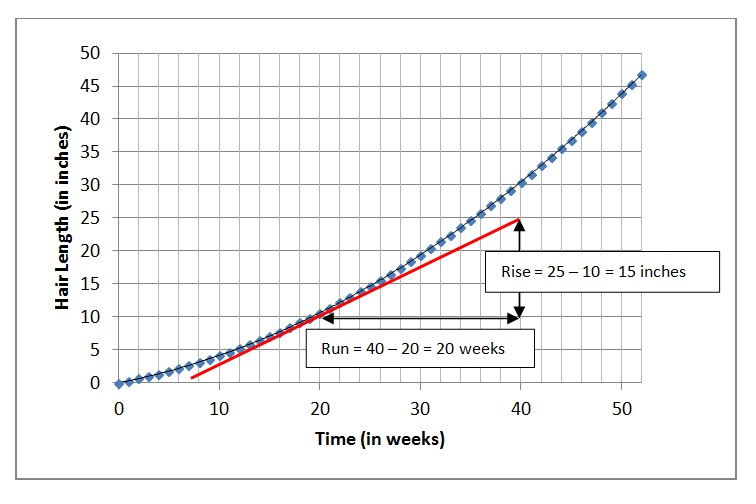After you draw the tangent line, you choose any two points on that line and use them to calculate rise over run.  So, using the values we get from the graph above, we calculate that the slope of the tangent line is (15 inches)/(20 weeks) = .75 inches/week. So now we know:

At week #20, the instantaneous velocity of hair growth was .75 inches/week.

2. WITH CALCULUS

The point of the entire discussion up until this point has really been to remind you about the idea of slope as a rate of change and to introduce (or re-introduce) what we mean by instantaneous rate of change.  But I don’t expect that we will be drawing many tangent lines by hand during AP Physics.  When we need to know the slope of a graph, there are formulas for that kind of thing.  We’ll start looking at those formulas in the next post.

# Even Hairier

In my last post, I reported on the results of your hair-growing experiment.

Now suppose that at the end of the year, you go back and analyze your year of hair growth.  You can see from the graph that your hair grew steadily at a rate of 0.3 inches per week.  That was the slope of the graph no matter what time interval you chose to examine.  So we concluded that the rate was constant.

If we feel like it, instead of graphing hair length, we could graph the graph the growth rate instead.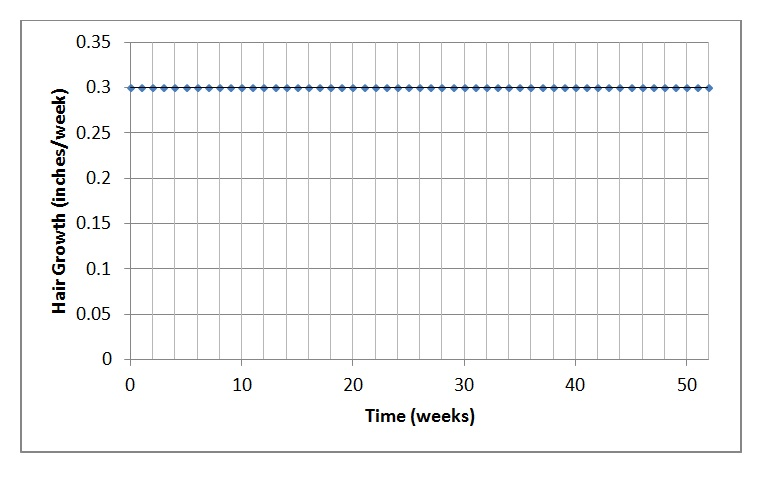This graph is not that exciting.  Since your hair grew at the same rate week after week, this graph is a horizontal line.  But it is important to understand that we are still talking about the same experiment.  The first graph showed hair length.  That graph slopes uphill at a constant rate.  This new graph shows growth rate – it is the slope of the previous graph.  And since that graph had a constant slope, this new graph is horizontal.

Now let’s suppose that you were not satisfied with the year’s hair growth.  So you search the internet for hair growth accelerators.  (I’m sure people actually do this.)  And let’s also pretend that you find one that really works as advertised.  It promises that if you use it every day, you will experience steadily increasing hair- growth-rates.  In fact, they promise:

Guaranteed Acceleration = .023 inches/week2

Exactly what does this mean? If you are new to physics, it is possible that you don’t know how to interpret this guarantee. I mean, what is a “week squared” anyway?

Still, you decide to try it.  In the interest of science, you shave off your hair on New Year’s Day and begin the experiment.  Here are your results: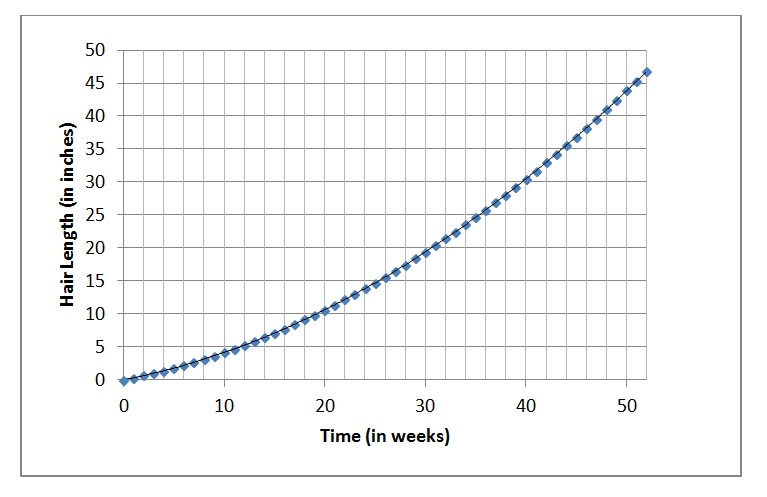Now that’s some seriously long hair!  In one year, your hair has grown nearly four feet!  And when we look at the graph of growth rate, it is now a little more interesting: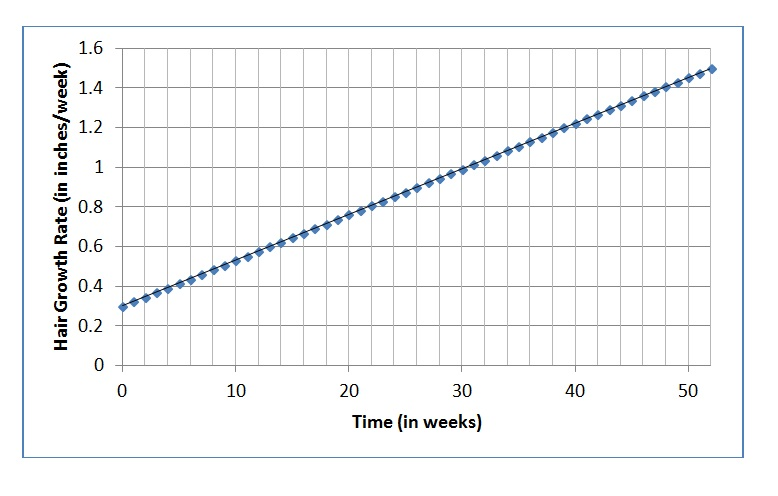Your rate of hair growth certainly increased.  It was 0.3 inches per week at the beginning of the year.  And by the end of the year, the rate had increased to 1.5 inches per week, increasing by 1.2 inches per week over the course of the year.  But the increase did not happen all at once. The growth rate increased steadily.

By how much did the growth rate increase each week?

We can answer this question two different ways.

1. Since we know that the rate increase for the entire year was 1.2 inches per week, we can divide to find the weekly increase:

1.2 ÷ 52 ≈ .023 inches per week per week

Let’s make sure to understand what we just figured out.  Each week, the growth rate increases by .023 inches per week.  At the beginning of the year, the rate was .3 inches per week.  After 1 week, it will have increased to .3 + .023 = .323 inches per week.  After 2 weeks, it will increase and additional .023 inches per week to .3 + .023 +.023 = .346 inches per week.  In other words:

Hair Growth Acceleration = .023 (inches/week)/week

When we are being lazy, we write:  .023 inches/week2

2. We can also find the growth rate by evaluating the slope of the graph of growth rate.  Just like hair “velocity” is the slope of the graph of hair length vs. time, hair acceleration is the slope of the velocity graph.  And since that graph is linear, we can pick any two points to calculate the rise over run.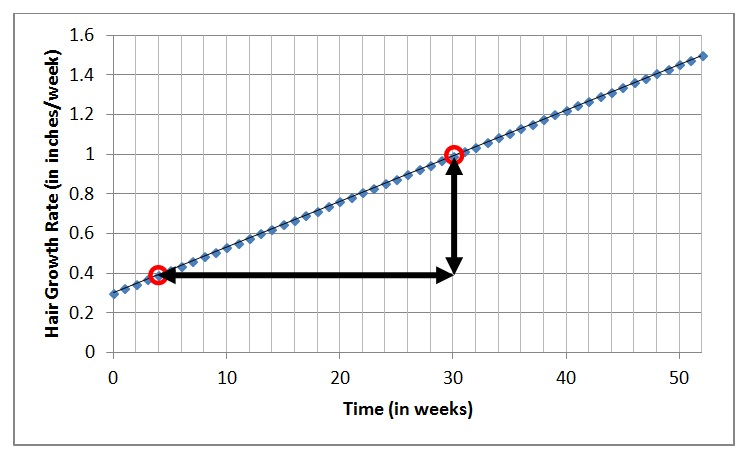Using the points I have chosen, we get a rise of .6 inches per week over a run of 26 weeks which comes out to a slope of .6 ÷ 26 ≈ .023 (inches/week)/week, just as we expected.

But to even draw a graph like this, we must somehow believe that at any given moment in time, your hair is growing at some particular rate.  So now let’s look back at the graph of hair length vs. time:You can see that this graph is NOT linear.  As time passes, the slope gets steeper because your hair growth is accelerating.  Every instant, the rate is changing.   Again, we seem to be talking about instantaneous growth rates.  That’s our next topic.

# Hairy Questions

The next bunch of posts are intended for my incoming AP-C Physics students.   The course is a calculus-based version of 1st-year college level physics.  Some of my students will be starting to study calculus just as they are starting this course.  These posts are designed to give them enough of an introduction to stay above water.  So here we go…

Slope is a rate of change.

Many of the quantities you have already encountered in your study of physics are rates of change of other quantities. For example:

Velocity is the rate of change of position with respect to time.

Acceleration is the rate of change of velocity with respect to time.

Force is the rate of change of momentum with respect to time.

Current is the rate of change of electric charge with respect to time.

Induced Voltage is the rate of change of magnetic flux with respect to time.

And there were many times when you found the rate of change by graphing the quantity vs. time and then evaluating the slope of the resulting line.  Let’s look at a non-physics example.

For one year, you let your hair grow, measuring the length at the end of every week.  You graph the results of your experiment below, along with the line of best fit.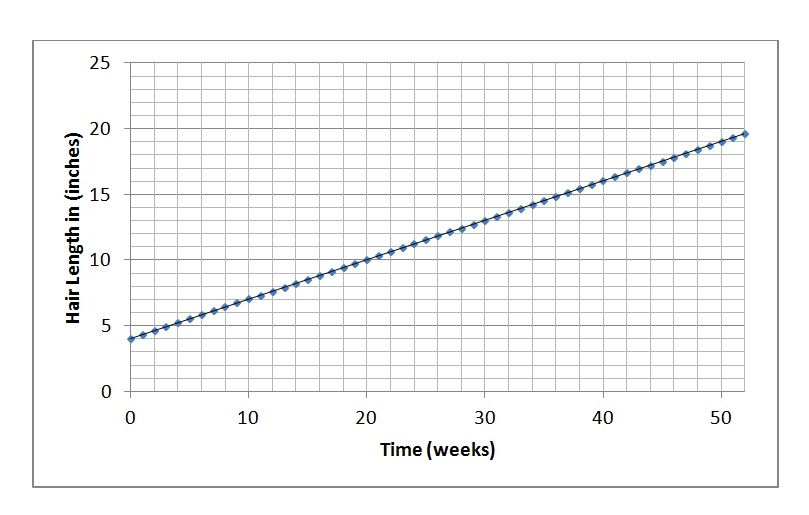There are some questions that we can answer by reading the information directly from this graph.  For example…

1. How long was your hair after week 10?

2. When did your hair reach a length of 15 inches?

3. How much longer did you hair grow between the end of week 20 and the end of week 30?

Then there are questions that you answer by finding the slope.

4. What was the average rate of hair growth over the first 5 weeks?

5. What was the average rate of hair growth between week 10 and week 20?

6. What was the average rate of hair growth for the entire year?

7. At what rate was your hair growing at the end of week 30?

Questions 4, 5 and 6 are all answered the same way: you find the rise over run to determine the rate of change.  This should remind you of your first weeks in physics class, studying kinematics.  We could even call this slope  the “hair velocity” if we like.

And in fact, you should get the same answer to all three questions.  The graph is linear.  You will find the same slope no matter what two points you pick.  We can say that your hair is growing at constant velocity.

Question 7 is different from the others in a subtle but important way.  It asks you to find the growth rate at a specific time – and not over a time interval!  It is asking for an instantaneous rate of change, not an average.  Fortunately, this time, it’s still an easy question.  Since we have seen that the growth is at a constant rate, it is reasonable to assume that the rate at any particular instant is the same as the average rate for the entire period (or in fact, any period you pick!  It doesn’t matter – the rate is constant.)

But while that gives us an answer, it also lets us side-step some important questions:

1.  How would we find the rate of change at a given time if your hair growth rate wasn’t constant?

2.  What do we even mean by the rate AT a given time?  Don’t you need to compare TWO times in order to find a rate of change?

We’ll consider these in the nest post when you experiment with some hair-growth-acceleration tonic you found on line.

# It Takes a Square…

…to Complete a Square

As you have seen, quadratic equations can pop up unexpectedly.  Here’s one:

x2 + 4x – 12 = 0

I chose this one because it does “factor”, but I don’t want to solve it that way.  Factoring causes stress.  When you have not yet found the factors, you start to wonder:

Is it just me? Or does this thing have no factors?

When you are ready to give up, you turn to the quadratic formula.  To solve:

ax2 + bx + c = 0

you just have to identify the values of a, b and c and then plug them into the magic formula:For our example, a=1, b=4 and c= -12.  So we get:But you have to admit, there’s something mindless and robot-like about solving quadratics this way.  It’s like we are impersonating a computer.  If you are going to be mindless, you might as well use the solve function on a TI-89 and be done with it.  But there is another alternative.  We could go back to the algorithm that the quadratic formula is derived from.  It’s clever and it has style.  And even better, it has diagrams!

COMPLETING THE SQUARE

Let’s start again with:

x2 + 4x – 12 = 0

And re-write it:

x2 + 4x = 12

And one more time:

x2 + 2x +2x = 12

But why do this?  Well, the left side of that equation has a nice picture that goes with it: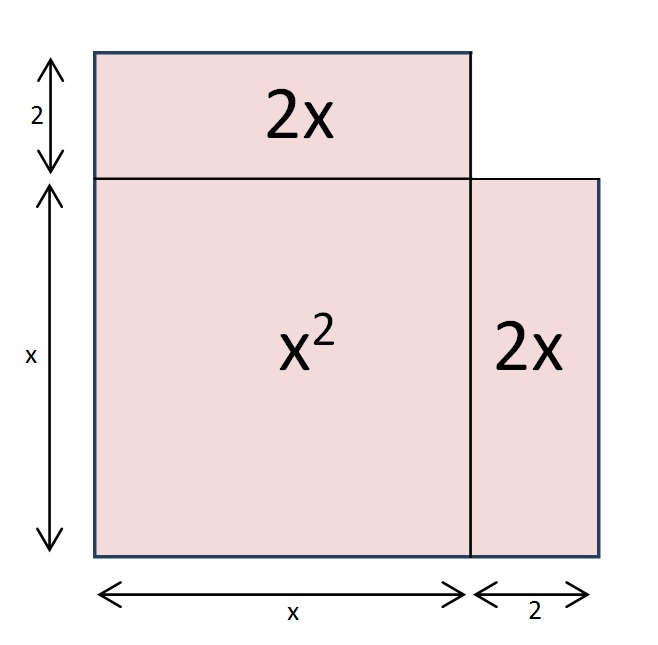It’s ALMOST a square.  And if we add one little square…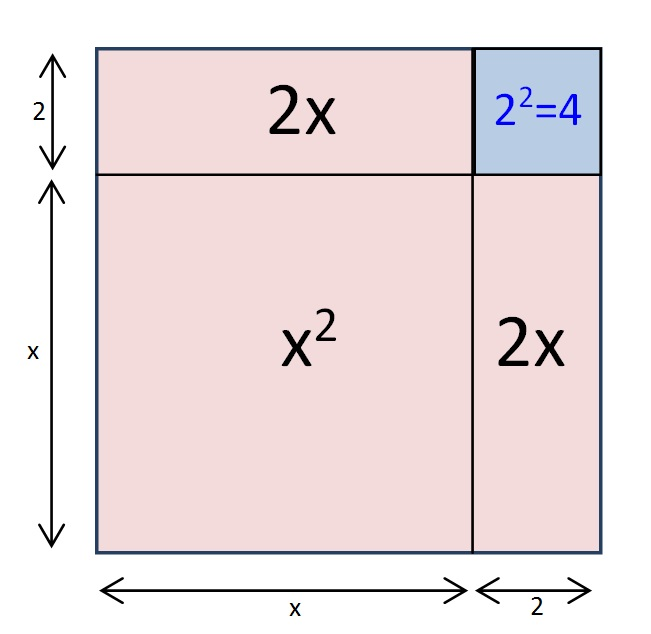We have just “completed the square”!

By adding that little blue square, we have made a bigger square with a side of length (x+2).

But that addition would leave our original equation out of balance.  So if we add 22 to one side, we also have to add it to the other side:

x2 + 2x +2x + 4 = 12 + 4

As you can see from the picture, the left side of our equation is now a perfect square. That means that we can write:

(x + 2)2 = 16

We can solve this pretty quickly.  Take the square root of both sides to get:

(x + 2) = ±4

x= -4 – 2 = -6 or 4 – 2 = 2

Q: Isn’t factoring faster?

A: It is when it is.  But when it isn’t, it really isn’t. And completing the square works either way.

For example, here’s one that doesn’t factor:

x2 + 5x – 4 = 0

x2 + 2.5x +2.5x = 4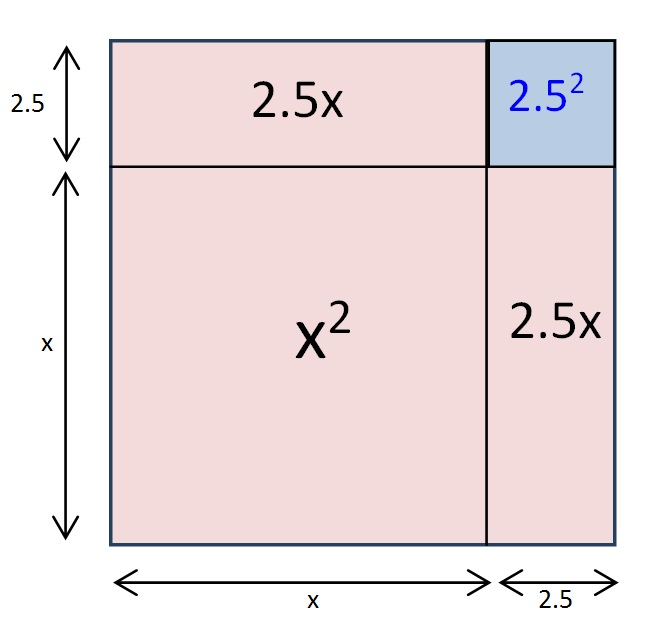x2 + 2.5x +2.5x + 2.52= 4 + 2.52

(x + 2.5)2 = 10.25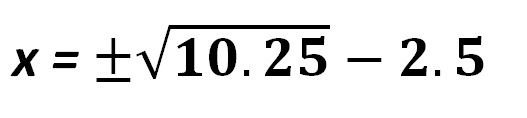You will still need a calculator if you want to tidy up, but you would have needed one had you used the quadratic formula instead.

OK, I am not expecting to win a lot of converts here.  I just think that when you connect it with its diagrams, completing the square has an elegance to it that the quadratic formula lacks.  (But if I had to do a whole page of these to do,say 1 – 99 odd, I would grab a TI-89 and move on.)

I have only seen one physics student use completing the square in class.  She did it effortlessly with the same automaticity that most students bring to “cross-multiply and divide”.  But she learned her algebra in another country.